# Pointer Basics

This document introduces the basics of pointers as they work in several computer languages -- C, C++, Java, and Pascal. This document is the companion document for the Pointer Fun with Binky digital video, or it may be used by itself.

• Section 1 -- The three basic rules of pointers
• Section 2 -- A simple code example (the same example used in the video)
• Section 3 -- Study questions with solutions

This is document 106 in the Stanford CS Education Library. This and other free materials are available at cslibrary.stanford.edu. Some documents that are related to this one include...

• Pointer Fun Video -- a silly 3 minute digital video on the basics of pointers. Designed to go with the document in front of you. (http://cslibrary.stanford.edu/104/)
• Pointers and Memory --a 31 page explanation of the common features and techniques for using pointers and memory in C and other languages. (http://cslibrary.stanford.edu/102/)

## Section 1 -- Pointer Rules

One of the nice things about pointers is that the rules which govern how they work are pretty simple. The rules can be layered together to get complex results, but the individual rules remain simple.

### 1) Pointers and Pointees

A pointer stores a reference to something. Unfortunately there is no fixed term for the thing that the pointer points to, and across different computer languages there is a wide variety of things that pointers point to. We use the term pointee for the thing that the pointer points to, and we stick to the basic properties of the pointer/pointee relationship which are true in all languages. The term "reference" means pretty much the same thing as "pointer" -- "reference" implies a more high-level discussion, while "pointer" implies the traditional compiled language implementation of pointers as addresses. For the basic pointer/pointee rules covered here, the terms are effectively equivalent.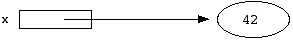The above drawing shows a pointer named `x` pointing to a pointee which is storing the value 42. A pointer is usually drawn as a box, and the reference it stores is drawn as an arrow starting in the box and leading to its pointee.

Allocating a pointer and allocating a pointee for it to point to are two separate steps. You can think of the pointer/pointee structure as operating at two levels. Both the levels must be set up for things to work. The most common error is concentrating on writing code which manipulates the pointer level, but forgetting to set up the pointee level. Sometimes pointer operations that do not touch the pointees are called "shallow" while operations on the pointees are called "deep".

### 2) Dereferencing

The dereference operation starts at the pointer and follows its arrow over to access its pointee. The goal may be to look at the pointee state or to change the pointee state.

The dereference operation on a pointer only works if the pointer has a pointee -- the pointee must be allocated and the pointer must be set to point to it. The most common error in pointer code is forgetting to set up the pointee. The most common runtime crash because of that error in the code is a failed dereference operation. In Java the incorrect dereference will be flagged politely by the runtime system. In compiled languages such as C, C++, and Pascal, the incorrect dereference will sometimes crash, and other times corrupt memory in some subtle, random way. Pointer bugs in compiled languages can be difficult to track down for this reason.

## Section 2 -- Binky's Code Example

This section presents the same code example used in the Pointer Fun With Binky video. There are versions of the code in several computer languages. All the versions have the same structure and demonstrate the same basic rules and lessons about pointers; they just vary in their syntax. Independent of any particular language, the basic structure of the example is...
 1. Allocate two pointers `x` and `y`. Allocating the pointers does not allocate any pointees.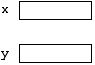2. Allocate a pointee and set `x` to point to it. Each language has its own syntax for this. What matters is that memory is dynamically allocated for one pointee, and `x` is set to point to that pointee.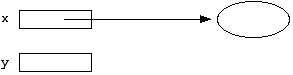3. Dereference `x` to store 42 in its pointee. This is a basic example of the dereference operation. Start at `x`, follow the arrow over to access its pointee.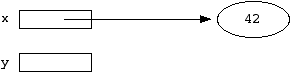4. Try to dereference `y` to store 13 in its pointee. This crashes because `y` does not have a pointee -- it was never assigned one.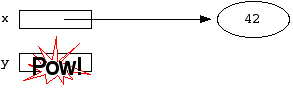5. Assign `y = x;` so that `y` points to `x`'s pointee. Now `x` and `y` point to the same pointee -- they are "sharing".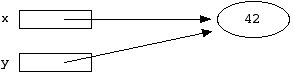6. Try to dereference `y` to store 13 in its pointee. This time it works, because the previous assignment gave `y` a pointee.### Versions

Below are versions of this example in C, Java, C++, and Pascal. They all do the same thing -- the syntax is just adjusted for each language.

### C Version

The pointers `x` and `y` are allocated as local variables. The type `int*` means "pointer which points to ints". As Binky learns, the pointers do not automatically get pointees. The pointee for `x` is dynamically allocated separately with the standard library function `malloc()`. The syntax `*x` dereferences x to access its pointee.

```void main() {
int*    x;  // Allocate the pointers x and y
int*    y;  // (but not the pointees)

x = malloc(sizeof(int));    // Allocate an int pointee,
// and set x to point to it

*x = 42;    // Dereference x to store 42 in its pointee

*y = 13;    // CRASH -- y does not have a pointee yet

y = x;      // Pointer assignment sets y to point to x's pointee

*y = 13;    // Dereference y to store 13 in its (shared) pointee
}
```

### Java Version

In Java, the most common pointer/pointee structure is a local variable pointer which points to a pointee object of some class. So in keeping with our plan to create a pointee which stores an integer, we define an `IntObj` class that stores one integer. We can then create an `IntObj` pointee to store the int. As Binky learns, allocating the pointer with code like `IntObj x;` does not automatically allocate the pointee. The `IntObj` pointee is allocated with a call to `new`. The syntax `x.value` dereferences `x` to access the `.value` field in its pointee.
```class IntObj {
public int value;
}

public class Binky() {
public static void main(String[] args) {
IntObj  x;  // Allocate the pointers x and y
IntObj  y;  // (but not the IntObj pointees)

x = new IntObj();   // Allocate an IntObj pointee
// and set x to point to it

x.value = 42;   // Dereference x to store 42 in its pointee

y.value = 13;   // CRASH -- y does not have a pointee yet

y = x;  // Pointer assignment sets y to point to x's pointee

y.value = 13;   // Deference y to store 13 in its (shared) pointee
}
}
```

### C++ Version

The only difference in this version from the C version above is that the standard operator `new` is used instead of `malloc()`.
```void main() {
int*    x;  // Allocate the pointers x and y
int*    y;  // (but not the pointees)

x = new int;    // Allocate an int pointee,
// and set x to point to it

*x = 42;    // Dereference x to store 42 in its pointee

*y = 13;    // CRASH -- y does not have a pointee yet

y = x;      // Pointer assignment sets y to point to x's pointee

*y = 13;    // Dereference y to store 13 in its (shared) pointee
}
```

### Pascal Version

This is structurally identical to the C version, but with Pascal syntax. The type `^Integer` means "pointer which points to integers". As Binky learns, allocating the pointer does not automatically allocate its pointee. The standard procedure `New()` takes a pointer argument, allocates a new pointee, and sets the pointer to point to it. The expression `x^` dereferences x to access its pointee.
```Procedure main
var x:^Integer; /* Allocate the pointers x and y */
var y:^Integer; /* (but not the pointees)        */
Begin
New(x); /* Allocate a pointee and set x to point to it */

x^ := 42;   /* Deference x to store 42 in its pointee */

y^ := 13;   /* CRASH -- y does not have a pointee yet */

y := x; 	/* Pointer assignment makes y point to x's pointee */

y^ := 13;   /* Dereference y to store 13 in its (shared) pointee */
End;
```

## Section 3 -- Study Questions

These study questions cover review basic features of pointers. Two of the questions make heavy use of memory drawings. Memory drawings are an excellent way to think through pointer problems.

### Question 1

At the end of the above code, `y` is set to have a pointee and then dereferenced it store the number 13 into its pointee. After this happens, what is the value of `x`'s pointee?

Answer: The value of `x`'s pointee is 13 because it is also `y`'s pointee. This is what sharing is all about -- multiple pointers pointing to one pointee.

### Question 2

Consider the following drawing...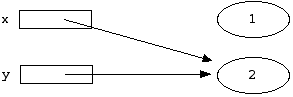Using the language of your choice, write some code that creates the above pointer structure.

1. Allocate two pointers.
2. Allocate two pointees and set the pointers to point to them.
3. Store the numbers 1 and 2 into the pointees.
4. Assign the first pointer to point to the second pointee. This "loses" the reference to the first pointee which is unusual, but that's what the question calls for.
C Code Java Code
```{
int* x;
int* y;

x = malloc(sizeof(int));
y = malloc(sizeof(int));

*x = 1;
*y = 2;

x = y;
}
```
```{
IntObj x;
IntObj y;

x = new IntObj();
y = new IntObj();

x.value = 1;
y.value = 2;

x = y;
}
```

### Question 3

Suppose you have a pointee type called "Node" which contains two things: an int, and a pointer to another Node (the declaration for such a Node type is given below). With such a pointee type, you could arrange three Node pointees in a structure where they were pointing to each other like this...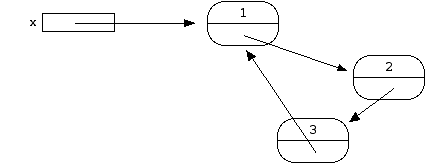The pointer named `x` points to the first Node pointee. The first Node contains a pointer to the second, the second contains a pointer to the third, and the third contains a pointer back to the first. This structure can be build using only the rules of pointee allocation, dereferencing, and assignment that we have seen. Using the declaration below, each Node contains an integer named `value` and a pointer to another Node named `next`.
C Code Java Code
```struct Node {
int value;
struct Node* next;
};
```
```class Node {
public int value;
public Node next;
};
```

Write the code to build the structure in the above drawing. For convenience, you may use temporary pointers in addition to `x`. The only new syntax required is that in C, the operator `->` dereferences a pointer to access a field in the pointee -- so `->value` accesses the field named `value` in `x`'s pointee.

1. Allocate three pointers: x for the first Node, and temporary pointers `y` and `z` for the other two Nodes.
2. Allocate three Node pointees and store references to them in the three pointers.
3. Dereference each pointer to store the appropriate number into the `value` field in its pointee.
4. Dereference each pointer to access the `.next` field in its pointee, and use pointer assignment to set the `.next` field to point to the appropriate Node.
C Code Java Code
```{
// Allocate the pointers
struct Node* x;
struct Node* y;
struct Node* z;

// Allocate the pointees
x = malloc(sizeof(Node));
y = malloc(sizeof(Node));
z = malloc(sizeof(Node));

// Put the numbers in the pointees
x->value = 1;
y->value = 2;
z->value = 3;

// Put the pointers in the pointees
x->next = y;
y->next = z;
z->next = x;
}
```
```{
// Allocate the pointers
Node x;
Node y;
Node z;

// Allocate the pointees
x = new Node();
y = new Node();
z = new Node();

// Put the numbers in the pointees
x.value = 1;
y.value = 2;
z.value = 3;

// Put the pointers in the pointees
x.next = y;
y.next = z;
z.next = x;
}
```

The Node structure introduced here is actually a real data type used to build the "linked list" data structure. Linked lists are a realistic applied use of pointers and are an excellent area to develop your pointer skills. See Linked List Basics and Linked List Problems in the Stanford CS Education Library for lots of linked list material.

### Postscript

Copyright Nick Parlante, 1999. This material may be copied and redistributed so long as the standard Stanford CS Education Library notice on the first page is retained: "This is document 106 in the Stanford CS Education Library. This and other free materials are available at cslibrary.stanford.edu."

I hope that you benefit from this material in the spirit of goodwill in which it is given. That someone seeking education should have the opportunity to find it.

Up to the CS Education Library Home